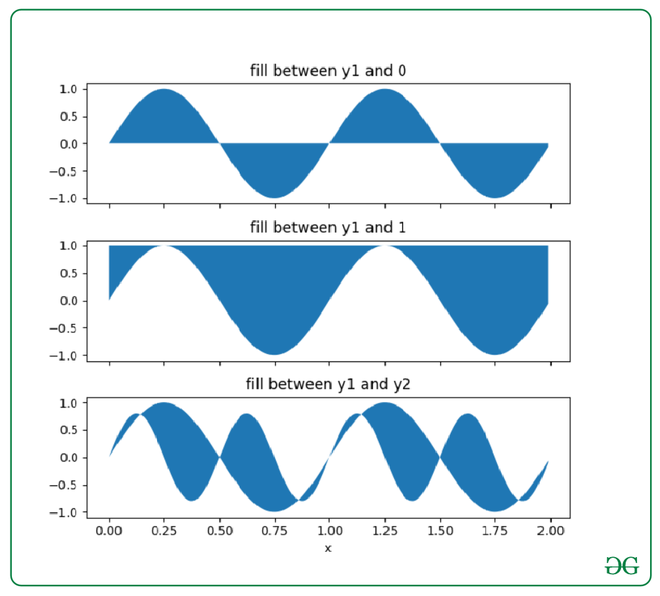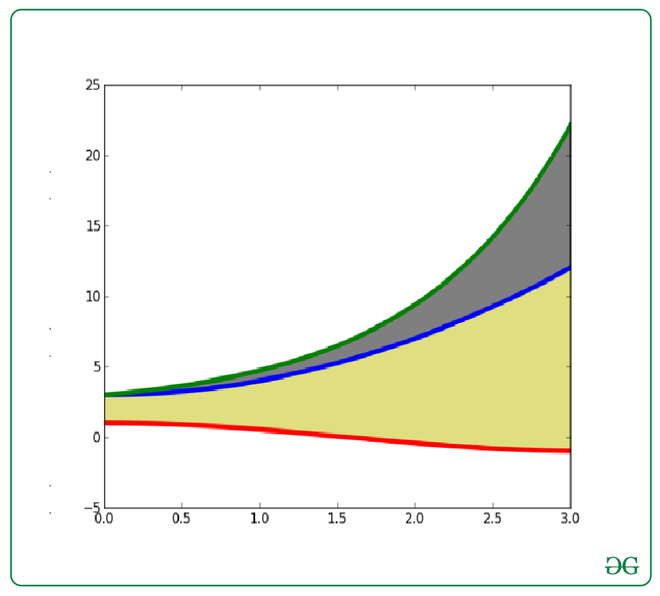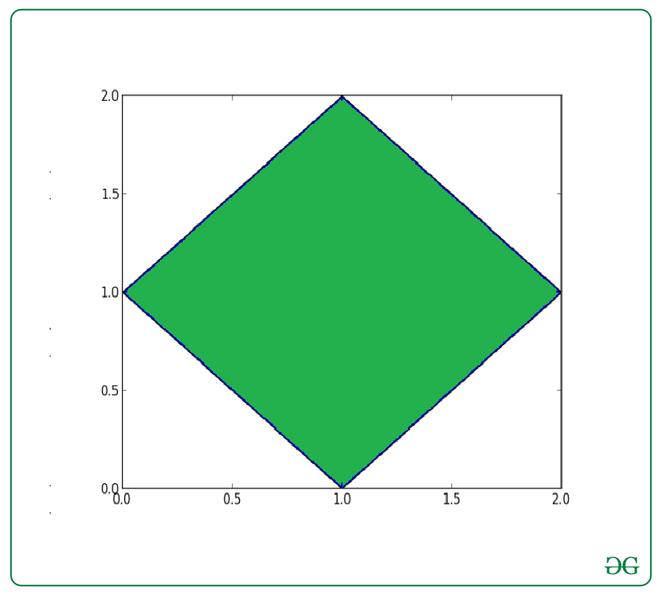GeeksforGeeks App
Open AppBrowser
Continue

# How to Fill Between Multiple Lines in Matplotlib?

With the use of the fill_between()  function in the Matplotlib library in Python, we can easily fill the color between any multiple lines or any two horizontal curves on a 2D plane.

Syntax: matplotlib.pyplot.fill_between(x, y1, y2=0, where=None, step=None, interpolate=False, *, data=None, **kwargs)

Example 1: Color between the curve of the mathematical function f(x)=sin(x)

## Python3

 `import` `pylab as plt``import` `numpy as np` `x ``=` `np.arange(``0.0``, ``2``, ``0.01``)``y1 ``=` `np.sin(``2` `*` `np.pi ``*` `x)``y2 ``=` `0.8` `*` `np.sin(``4` `*` `np.pi ``*` `x)` `fig, (ax1, ax2, ax3) ``=` `plt.subplots(``  ``3``, ``1``, sharex``=``True``, figsize``=``(``6``, ``6``))` `ax1.fill_between(x, y1)``ax1.set_title(``'fill between y1 and 0'``)` `ax2.fill_between(x, y1, ``1``)``ax2.set_title(``'fill between y1 and 1'``)` `ax3.fill_between(x, y1, y2)``ax3.set_title(``'fill between y1 and y2'``)``ax3.set_xlabel(``'x'``)``fig.tight_layout()`

Output:Example 2: Color between the curve of the mathematical function f(x)=cos(x) and f(x)=exp(x) :-

## Python3

 `import` `pylab as plt``import` `numpy as np` `X ``=` `np.linspace(``0``, ``3``, ``200``)``Y1 ``=` `X``*``*``2` `+` `3``Y2 ``=` `np.sin(X)``Y3 ``=` `np.cos(X)` `plt.plot(X, Y1, lw``=``4``)``plt.plot(X, Y2, lw``=``4``)``plt.plot(X, Y3, lw``=``4``)` `plt.fill_between(X, Y1, Y2, color``=``'k'``, alpha``=``.``5``)``plt.fill_between(X, Y1, Y3, color``=``'y'``, alpha``=``.``5``)` `plt.show()`

Output:Example 3: Color the Rhombus :-

## Python3

 `import` `matplotlib.pyplot as plt`  `x ``=` `[``1``, ``2``, ``1``, ``0``]``y ``=` `[``2``, ``1``, ``0``, ``1``]` `plt.fill(x, y)``plt.show()`

Output:My Personal Notes arrow_drop_up